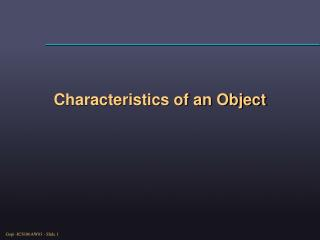DownloadDownload PresentationCharacteristics of an Object

# Characteristics of an Object

Download Presentation## Characteristics of an Object

- - - - - - - - - - - - - - - - - - - - - - - - - - - E N D - - - - - - - - - - - - - - - - - - - - - - - - - - -
##### Presentation Transcript

1. Characteristics of an Object

2. Object Characteristics • Topological properties • Manifolds (w/ boundaries) /Non-manifold • Euler characteristic/Genus/Betti numbers/ • Orientability • Homeomorphism/ Homology groups etc. • Geometric properties • Curvature, continuity • Surface parameterization • Convexity and concavity • Silhouette visibility

3. (Layman) Manifold Definitions • Manifolds • 2D: Every edge has exactly two incident triangles. • 3D: Every triangle has exactly two incident tetrahedrons. • Manifolds with boundaries • 2D: Every edge has either one or two incident triangles. • 3D: Every triangle has either one or two incident tetrahedrons. • Non-manifolds • That does not have the above restrictions.

4. (Expert) Manifold Definitions • Manifolds • 2D: Neighborhood of every point belonging to the object is homeomorphic to an open disc. • 3D: Neighborhood of every point belonging to the object is homeomorphic to an open ball. • Manifolds with boundaries • 2D: …(as above) or a half-disk. • 3D:…(as above) or a half-ball • Non-manifolds • That does not have the above restrictions.

5. Genus (g) of a manifold • Applicable only for manifolds • (Naïve) Number of “handles”. • Sphere has g=0; cube has g=0; torus has g=1; coffee cup has g=1.

6. Euler Characteristic (e) of a manifold • e = V-E+F (V: Vertices, E: Edges, F: Faces). • Applicable only for manifolds • In general • e=(0 dim)-(1 dim)+(2 dim)-(3 dim)+(4 dim)… • Relationship between e and g: e=2-2g • Sphere or Cube: e=2-2(0)=2 • Torus: e=2-2(1)=0 • Verify: Cube has 8 vertices, 12 edges, 6 faces • e = V-E+F = 8-12+6 = 2

7. Orientability of an object • If you have consistent normal direction for a point then the object is orientable. Otherwise, non-orientable. Mobius Strip

8. In this course.. • 2D orientable manifolds with boundaries. Remember: manifolds with boundaries is a superset of manifolds, and non-manifold is a superset of manifolds with boundaries. Non-manifold actually means that “need not” be a manifold; not “is not” a manifold.